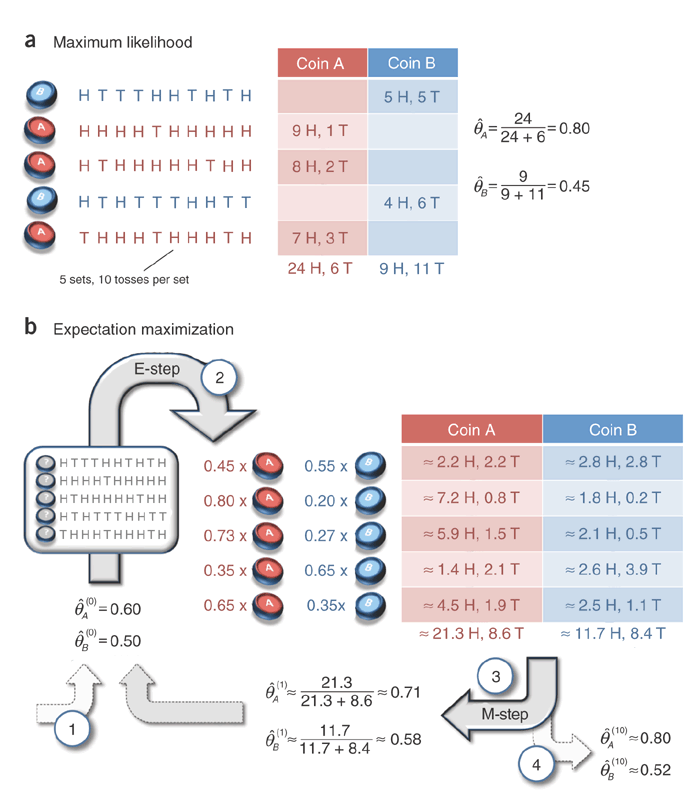## Expectation Maximisation with Python : Coin Toss¶

This notebook implements the example, I consider a classic for understanding Expectation Maximisation.

Notations:

\begin{align*} \theta_A &= \text{Probability of a Heads showing up given the coin tossed is A}\ \theta_B &= \text{Probability of a Heads showing up given the coin tossed is B}\ \end{align*}
In :
%matplotlib notebook
from __future__ import division
from collections import OrderedDict
from scipy.stats import binom as binomial
import numpy as np
import matplotlib.pyplot as plt
import seaborn as sns
#from ipywidgets import StaticInteract, RangeWidget
import pandas as pd
from IPython.display import display, Image
from scipy.spatial.distance import euclidean
from sympy import init_printing, symbols, Eq
init_printing()

In :
Image('images/nbt1406-F1.png')

Out:In :
coin_toss = []
coin_toss.append('H T T T H H T H T H'.split())
coin_toss.append('H H H H T H H H H H'.split())
coin_toss.append('H T H H H H H T H H'.split())
coin_toss.append('H T H T T T H H T T'.split())
coin_toss.append('T H H H T H H H T H'.split())

In :
columns = range(1,11)
df = pd.DataFrame(coin_toss, index=None, columns=columns)
df.index.rename('Toss')

Out:
Int64Index([0, 1, 2, 3, 4], dtype='int64', name=u'Toss')

Our configuration looks like this:

In :
df

Out:
1 2 3 4 5 6 7 8 9 10
0 H T T T H H T H T H
1 H H H H T H H H H H
2 H T H H H H H T H H
3 H T H T T T H H T T
4 T H H H T H H H T H

## Case 1: Identity of coin being tossed known¶

If the identity of the coin being tossed is known and is observed = ['B', 'A', 'A', 'B', 'A'] it is not so difficult to calculate the corresponding values of $\theta_A$ and $\theta_B$:

$$\theta_A = \frac{\text{Total Heads when coin tossed is A}}{\text{Total tosses for coin A}}$$$$\theta_B = \frac{\text{Total Heads when coin tossed is B}}{\text{Total tosses for coin B}}$$
In :
thetaA, thetaB = symbols('theta_A theta_B')
a,b = thetaA, thetaB # Hack to display

In :
## Observed Case

observed = ['B', 'A', 'A', 'B', 'A']
index_A = [i for i,x in enumerate(observed) if x=='A']
index_B = [i for i,x in enumerate(observed) if x=='B']

total_tosses = df.size

A_tosses = df.iloc[index_A].unstack()
B_tosses = df.iloc[index_B].unstack()


In :
(a, theta_A)

Out:
$$\left ( \theta_{A}, \quad 0.8\right )$$
In :
(b, theta_B)

Out:
$$\left ( \theta_{B}, \quad 0.45\right )$$

## Case 2 Identity of coin being tossed is unknown¶

When the identity of coin being tossed is unknwon we rely on Expectation Maximisation to give us the estimates of $\theta_A$ and $\theta_B$. We start with an initial value of $\theta_A, \theta_B$ and then given the observed data (the 50 coin tosses) run the ‘E-step’ calculating the probability of coin $A$ or $B$ being used for a series of tosses(Remember each set of 10 coin tosses is done using

In :
thetaA = 0.6
thetaB = 0.5

def em(theta_old):
row_prob = []
## Expectation
for row in coin_toss:
p_t = p_a+p_b
p_a = p_a/p_t
p_b = p_b/p_t

## Maximisation
new_coin_toss = []
for row in row_prob:
total_tosses = row['total_tosses']
new_theta = OrderedDict({'A': new_pa, 'B': new_pb})
display(df)
return new_theta

theta = OrderedDict({'A': thetaA, 'B': thetaB})

In :
theta_new = OrderedDict()
max_iterations = 10000
iterations = 0
diff = 1
tolerance = 1e-6
while (iterations < max_iterations) and (diff>tolerance):
new_theta = em(theta)
diff = euclidean(new_theta.values(), theta.values())
theta = new_theta

0 2.245745 2.245745 2.754255 2.754255
1 7.244870 0.804986 1.755130 0.195014
2 5.867737 1.466934 2.132263 0.533066
3 1.408625 2.112937 2.591375 3.887063
4 4.530506 1.941645 2.469494 1.058355
0 1.479097 1.479097 3.520903 3.520903
1 7.303594 0.811510 1.696406 0.188490
2 5.651376 1.412844 2.348624 0.587156
3 0.760578 1.140867 3.239422 4.859133
4 4.014738 1.720602 2.985262 1.279398
0 1.087962 1.087962 3.912038 3.912038
1 7.828637 0.869849 1.171363 0.130151
2 6.009233 1.502308 1.990767 0.497692
3 0.446362 0.669544 3.553638 5.330456
4 4.038084 1.730607 2.961916 1.269393
0 0.808513 0.808513 4.191487 4.191487
1 8.216144 0.912905 0.783856 0.087095
2 6.354109 1.588527 1.645891 0.411473
3 0.265334 0.398001 3.734666 5.601999
4 4.109732 1.761314 2.890268 1.238686
0 0.645102 0.645102 4.354898 4.354898
1 8.418405 0.935378 0.581595 0.064622
2 6.572406 1.643101 1.427594 0.356899
3 0.179981 0.269971 3.820019 5.730029
4 4.159435 1.782615 2.840565 1.217385
0 0.567711 0.567711 4.432289 4.432289
1 8.507517 0.945280 0.492483 0.054720
2 6.681854 1.670464 1.318146 0.329536
3 0.144896 0.217344 3.855104 5.782656
4 4.185923 1.793967 2.814077 1.206033
0 0.535440 0.535440 4.464560 4.464560
1 8.544022 0.949336 0.455978 0.050664
2 6.730149 1.682537 1.269851 0.317463
3 0.131238 0.196856 3.868762 5.803144
4 4.198972 1.799559 2.801028 1.200441
0 0.522786 0.522786 4.477214 4.477214
1 8.558587 0.950954 0.441413 0.049046
2 6.750249 1.687562 1.249751 0.312438
3 0.126013 0.189019 3.873987 5.810981
4 4.205202 1.802230 2.794798 1.197770
0 0.517957 0.517957 4.482043 4.482043
1 8.564351 0.951595 0.435649 0.048405
2 6.758425 1.689606 1.241575 0.310394
3 0.124026 0.186039 3.875974 5.813961
4 4.208106 1.803474 2.791894 1.196526
0 0.516135 0.516135 4.483865 4.483865
1 8.566629 0.951848 0.433371 0.048152
2 6.761725 1.690431 1.238275 0.309569
3 0.123272 0.184909 3.876728 5.815091
4 4.209430 1.804042 2.790570 1.195958
0 0.515451 0.515451 4.484549 4.484549
1 8.567530 0.951948 0.432470 0.048052
2 6.763053 1.690763 1.236947 0.309237
3 0.122987 0.184480 3.877013 5.815520
4 4.210025 1.804296 2.789975 1.195704
0 0.515196 0.515196 4.484804 4.484804
1 8.567887 0.951987 0.432113 0.048013
2 6.763588 1.690897 1.236412 0.309103
3 0.122879 0.184318 3.877121 5.815682
4 4.210288 1.804409 2.789712 1.195591
0 0.515100 0.515100 4.484900 4.484900
1 8.568028 0.952003 0.431972 0.047997
2 6.763804 1.690951 1.236196 0.309049
3 0.122838 0.184256 3.877162 5.815744
4 4.210403 1.804458 2.789597 1.195542
0 0.515065 0.515065 4.484935 4.484935
1 8.568084 0.952009 0.431916 0.047991
2 6.763891 1.690973 1.236109 0.309027
3 0.122822 0.184233 3.877178 5.815767
4 4.210453 1.804480 2.789547 1.195520
0 0.515051 0.515051 4.484949 4.484949
1 8.568106 0.952012 0.431894 0.047988
2 6.763926 1.690982 1.236074 0.309018
3 0.122816 0.184224 3.877184 5.815776
4 4.210474 1.804489 2.789526 1.195511
0 0.515046 0.515046 4.484954 4.484954
1 8.568115 0.952013 0.431885 0.047987
2 6.763940 1.690985 1.236060 0.309015
3 0.122814 0.184221 3.877186 5.815779
4 4.210483 1.804493 2.789517 1.195507
In :
(a, new_theta['A'])

Out:
$$\left ( \theta_{A}, \quad 0.796788954444\right )$$
In :
(b, new_theta['B'])

Out:
$$\left ( \theta_{B}, \quad 0.51958345063\right )$$
In [ ]:



Saket Choudhary is a PhD candidate at the University of Southern California